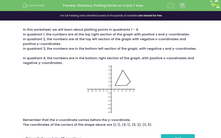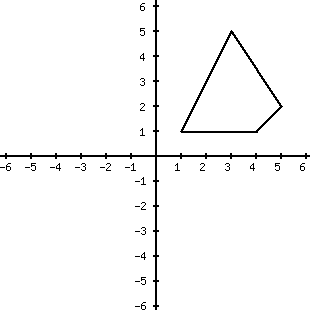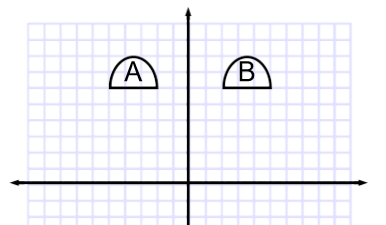# Statistics: Plotting Points on X and Y Axes

In this worksheet, students plot and read points and understand the simple reflection of points in the x- and y-axes.Key stage:  KS 2

Curriculum topic:   Statistics

Curriculum subtopic:   Use Charts and Graphs

Difficulty level:#### Worksheet Overview

In this worksheet, we will learn about plotting points in quadrants 1 - 4.

In quadrant 1, the numbers are at the top right section of the graph with positive x and y-coordinates.

In quadrant 2, the numbers are at the top left section of the graph with negative x-coordinates and positive y-coordinates.

In quadrant 3, the numbers are in the bottom left section of the graph, with negative x and y-coordinates.

In quadrant 4, the numbers are in the bottom right section of the graph, with positive x-coordinates and negative y-coordinates.Remember that the x-coordinate comes before the y-coordinate.

The coordinates of the corners of the shape above are (1, 1), (4, 1), (5, 2), (3, 5).

When we reflect a shape, it is mirrored across the x- or y-axis.

In the example below, the semi-circle (shape A) has been reflected across the y-axis to become shape B.

Notice how it is an equal distance from the y-axis on both sides.### What is EdPlace?

We're your National Curriculum aligned online education content provider helping each child succeed in English, maths and science from year 1 to GCSE. With an EdPlace account you’ll be able to track and measure progress, helping each child achieve their best. We build confidence and attainment by personalising each child’s learning at a level that suits them.

Get started